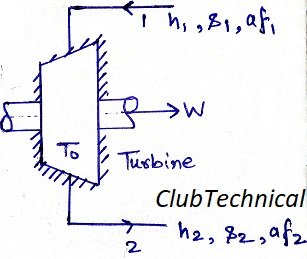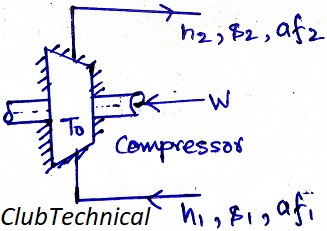# Second Law Efficiency [Turbine and Compressor]

By | March 16, 2019

## Second law efficiency

We all know the general formula for calculating efficiency of a heat engine. It is a ratio of output work to input heat.

This formula for calculating efficiency is in line with first law of thermodynamics. Which deals with the conservation of quantity of energy (not quality).

But when it comes to the second law of thermodynamics which deals with the quality of energy, a different formula for calculating efficiency is required.

This formula takes available energy into account. This efficiency is known as Second Law Efficiency.

Second law efficiency is the ratio of minimum exergy intake to perform given task to the actual exergy intake to perform the same task.

ηII = Minimum exergy intake to perform given task/ Actual exergy intake to perform the same task

Now let’s discuss the second law efficiency for turbine and compressor.

Where:

h: Enthalpy

af: Availability (exergy) of a flowing stream (or specific flowability)

s: Entropy

T0: Operating temperature of turbine

Note: In this calculation we are neglecting changes in kinetic and potential energies.

### Second law efficiency of an adiabatic turbine

Let us consider an adiabatic turbine (as shown in picture).From steady flow energy equation (SFEE).

∂W/∂m = h1 -h2   … (1)

We know

af = h – (T * s)

hence

af2 = h2 – (T0 * s2)

af1 = h1 – (T0 * s1)

Difference in specific flowability at inlet and outlet

af1 – af2 = h1 – h2 + T0 (s2 – s1)   … (2)

from equation (1) and (2)

af1 – af2 = (∂W/∂m) + T0 (s2 – s1)   … (3) (Maximum exergy intake)

For an ideal or reversible process

s2 – s1 = 0

Hence for a reversible process equation (3) becomes

af1 – af2 = ∂W/∂m (Minimum exergy intake)

Now, second law efficiency is

ηII = Minimum exergy intake to perform given task/ Actual exergy intake to perform the same task

ηII = (∂W/∂m)/ [(∂W/∂m) + T0 (s2 – s1)]

### Second law efficiency of an adiabatic compressor

Let us consider an adiabatic compressor (as shown in picture).From steady flow energy equation (SFEE).

∂W/∂m = h2 -h1 … (4)

af2 = h2 – (T0 * s2)

af1 = h1 – (T0 * s1)

Difference in specific flowability at inlet and outlet

af2 – af1 = h2 – h1 – T0 (s2 – s1)   … (5)

From equation (4) and (5)

af2 – af1 = (∂W/∂m) – T0 (s2 – s1)   … (6) (Maximum exergy intake)

For an ideal or reversible process

s2 – s1 = 0

Hence for a reversible process equation (6) becomes

af2 – af1 = ∂W/∂m (Minimum exergy intake)

Now, second law efficiency is

ηII = Minimum exergy intake to perform given task/ Actual exergy intake to perform the same task

ηII = (∂W/∂m)/ [(∂W/∂m) – T0 (s2 – s1)]

This site uses Akismet to reduce spam. Learn how your comment data is processed.